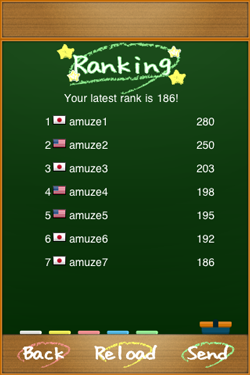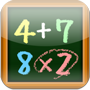# PluMul

たして★かけて
 Categoly：GamePrice:Free Add and multiply to make the same as a specific number. Can you work out as calculated? [How to play] Tap arranged numbers to add. Make the same as the number in the question box. - Any calculation formulas are OK to make the same as the specific number. Add up! Ex. 6 = 6 　　　　= 2 + 4 　　　　= 2 + 2 + 2 Just adding is not fun. Press [x2] to multiply! - Tap [x2] to double the numbers you choose. Ex. 10 = (3 + 2) [x2] When you make a wrong choice. - Tap a chosen number again to cancel. - If the answer of your calculation formula is higher than the number in the question box, your math formula will be reset. Choose numbers again! [LEVEL UP ＆ GAMEOVER] - It’s correct that the answer of your calculation formula becomes the same as the number in the question box. Go on to the next question. - If you give correct answers for six consecutive times, you will go to the next stage. - The arrangement of numbers changes every stage. - The more stages you pass, the shorter allotted time you have. - When limited time runs out, it’s game over.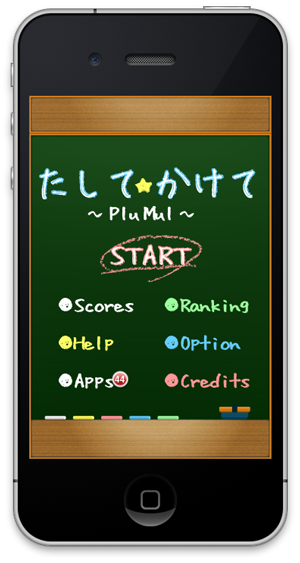Can you work out as calculated?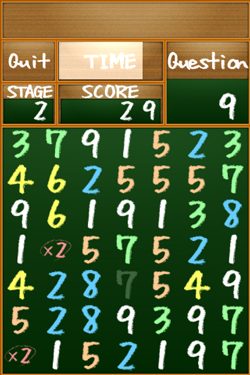You will go to the next stage!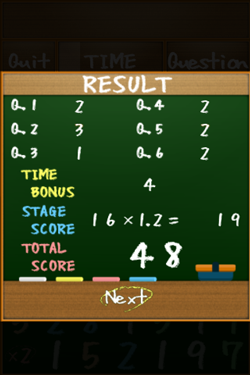Get a high ranking!